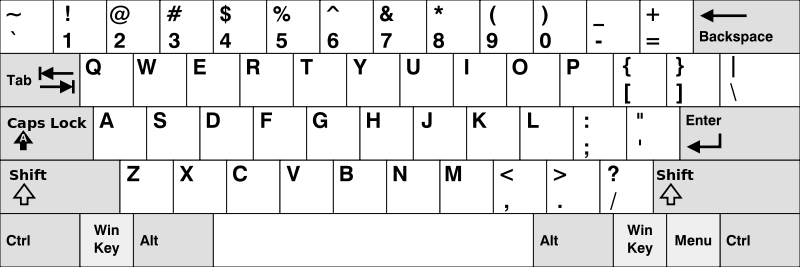### LeetCode• ㊗️
• 大家
• offer
• 多多！

## Problem

Given an array of strings words, return the words that can be typed using letters of the alphabet on only one row of American keyboard like the image below.

In the American keyboard:

• the first row consists of the characters “qwertyuiop”,
• the second row consists of the characters “asdfghjkl”, and
• the third row consists of the characters “zxcvbnm”.Example 1:

Input: words = ["Hello","Alaska","Dad","Peace"]


Example 2:

Input: words = ["omk"]
Output: []


Example 3:

Input: words = ["adsdf","sfd"]


Constraints:

• 1 <= words.length <= 20
• 1 <= words[i].length <= 100
• words[i] consists of English letters (both lowercase and uppercase).

## Code

class Solution {
public String[] findWords(String[] words) {
String row1 = "qwertyuiop";
String row2 = "asdfghjkl";
String row3 = "zxcvbnm";

HashMap<Character, Integer> map = new HashMap<>();

for (char c : row1.toCharArray()) {
map.put(c, 1);
}

for (char c : row2.toCharArray()) {
map.put(c, 2);
}

for (char c : row3.toCharArray()) {
map.put(c, 3);
}

List<String> list = new ArrayList<>();

for (String word : words) {
if (isValid(word, map)) {
}
}

String[] res = new String[list.size()];
for (int i = 0; i < list.size(); i++) {
res[i] = list.get(i);
}

return res;
}

private boolean isValid(String s, HashMap<Character, Integer> map) {
s = s.toLowerCase();
int index = map.get(s.charAt(0));

for (int i = 1; i < s.length(); i++) {
char c = s.charAt(i);
if (map.get(c) != index) {
return false;
}
}

return true;
}
}